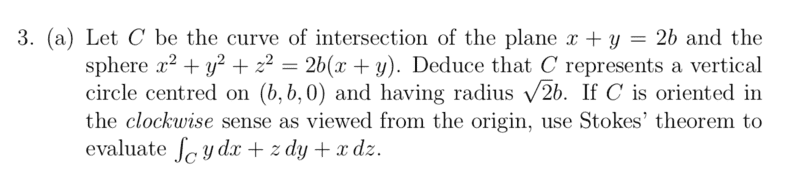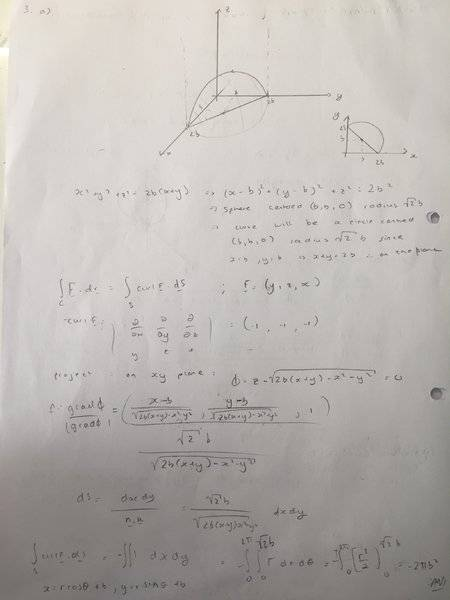# Using Stoke's theorem on an off-centre sphere

• Morbidly_Green
Yes. It doesn't matter what surface is bounded by the curve. No matter which surface you use, its surface integral is equal to the same ##\int_C \vec F\cdot dr##, so they are all equal. So you can evaluate the surface integral over simplest such surface, which is the planar disk.

## Homework Statement## Homework Equations

Stokes theorem
$$\int_C \textbf{F} . \textbf{dr} = \int_S \nabla \times \textbf{F} . \textbf{ds}$$

## The Attempt at a SolutionI have the answer to the problem but mine is missing a factor of$$\sqrt 2$$ I can't seem to find my error

#### Attachments

Apologies for that:

F= (y,z,x)
curl F = (-1,-1,-1)

Projecting the surface on the x-y plane:

Take ##\Phi = z- \sqrt{2b(x+y)-x^2 -y^2} = 0## from the equation of the surface to find the normal n. Then $$\textbf{n} = \dfrac{\nabla\Phi}{|\nabla\Phi|} = \dfrac{\left(\dfrac{x-b}{\sqrt{2b(x+y)-x^2 -y^2}} , \dfrac{y-b}{\sqrt{2b(x+y)-x^2 -y^2}},1\right)}{\dfrac{\sqrt{2}b}{\sqrt{2b(x+y)-x^2 -y^2}}}$$

Now ##ds = \dfrac{dx dy}{\textbf{n}.\textbf{k}} = \dfrac{\sqrt{2}b}{\sqrt{2b(x+y)-x^2 -y^2}} dxdy ##

$$\therefore \int_{S}\nabla \times \textbf{F}.\textbf{ds} = -\int\int dxdy = -\int^{2\pi}_{0}\int^{\sqrt{2}b}_{0} r \ dr d\theta = -2\pi b^2$$

Where for the change to polar coordinates was taken as ##x=rcos\theta +b , y=rsin\theta +b##

The plane ##x + y +0z= 2b## has unit normal ##\hat n = \frac 1 {\sqrt 2}\langle 1,1,0\rangle## in the correct direction. So $$\oint_C \vec F\cdot dr = \iint_S \langle -1,-1,-1\rangle \cdot \frac 1 {\sqrt 2}\langle 1,1,0\rangle ~dS =-\frac 2 {\sqrt 2} \cdot \text{Area of circle}$$
You don't need to jump through all those hoops to get the unit normal to a plane nor work the area integral.
Edit, added: What's worse, the plane is vertical and can't be expressed as a function of ##x## and ##y##.

LCKurtz said:
The plane ##x + y +0z= 2b## has unit normal ##\hat n = \frac 1 {\sqrt 2}\langle 1,1,0\rangle## in the correct direction. So $$\oint_C \vec F\cdot dr = \iint_S \langle -1,-1,-1\rangle \cdot \frac 1 {\sqrt 2}\langle 1,1,0\rangle ~dS =-\frac 2 {\sqrt 2} \cdot \text{Area of circle}$$
You don't need to jump through all those hoops to get the unit normal to a plane nor work the area integral.
Edit, added: What's worse, the plane is vertical and can't be expressed as a function of ##x## and ##y##.

So this is taking the surface to be the circle ? Thats much simpler then, thanks!

Morbidly_Green said:
So this is taking the surface to be the circle ? Thats much simpler then, thanks!
Yes. It doesn't matter what surface is bounded by the curve. No matter which surface you use, its surface integral is equal to the same ##\int_C \vec F\cdot dr##, so they are all equal. So you can evaluate the surface integral over simplest such surface, which is the planar disk.